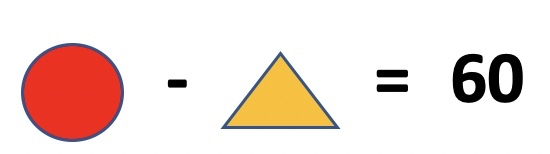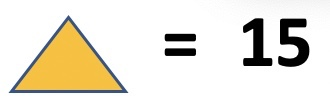### Olympiad Math Preparation – Class 2 -Subtraction of 2 and 3 digit numbers ( without regrouping/borrow )

1. The difference between greatest and smallest number is _________________ . Numbers : 178, 608, 578, 801, 999

2. Select the correct option

3. The difference of numbers shown in two abacuses below is ________________

4. Ifandthen what is value of?

5. Ifis subtracted from 100, the result is 45. Thenis _________.

6. What is difference of largest and smallest 3 digit number ?

7. Duggu has 85 chocolates. Dakshu has 24 chocolates. How many more chocolates Duggu has than Dakshu ?

8.and. Find the value ofRELATED POSTS :

# Olympiad Math Preparation – Class 2

## Addition of 2 and 3 digit numbers ( without regrouping/carry )

1. The sum of greatest and smallest number is _________________ . Numbers : 188, 508, 345, 801, 290

2. Select the correct option

3. The sum of numbers shown in two abacuses below is ________________

4. Ifandthen what is value of?

5. Ifis added to 100, the result is 150. Thenis _________.

6. What is sum of largest and smallest 3 digit number ?

7. Duggu has 54 chocolates. Dakshu has 45 chocolates. How many chocolates both of them have ?

8.and. Find the value ofRELATED POSTS :

# Olympiad Math Preparation – Class 2

## Expanded Form

1. 8 hundred + 5 tens + 2 ones is an even number ?

2. 5 hundred + 5 tens + 7 ones is an even number ?

3. The next odd number which comes after 6 hundred + 2 tens + 3 ones __________________

4. The next even number which comes after 3 hundred + 9 tens + 8 ones is _____________________

5. 4 hundred + 8 tens = _________________________

6. Expanded form of 346

7. Which abacus represents 7 hundred + 3 tens + 2 ones ?

8. Which option is correct ?

9. Duggu has 55 balls and Dakshu has 47 balls . What is total number of balls in expanded form ?

RELATED POSTS :

# Olympiad Math Preparation – Class 2

## Even and Odd Numbers

1. Which number is an even number ?

2. Which number is an odd number ?

3. The next odd number which comes after 145 is __________________

4. The next even number which comes after 98 is _____________________

5. Find the even number in the list of numbers: 145, 181, 192, 99, 177

6. Find the odd number in the list of numbers: 100, 78, 124, 191, 136

7. Which abacus shows odd number ?

8. Which option is correct ?

9. Which number does not belong to the group of numbers : 98, 114, 778, 277, 88, 190

10. Duggu has 54 chocolates and Dakshu has 47 chocolates. Who has odd number of chocolates ?

RELATED POSTS :

# Olympiad Math Preparation – Class 2

## Abacus and Place Value

1. The place value of 8 in 687 is ____________________

2. The place value of 9 in 975 is ____________________

3. The place value of 4 in 564 is ____________________

4. 2 in 925 is at ________________ place.

5. In 845, the number 8 is at ____________________ place.

6. 6 in 216 is at ________________ place.

7. Which abacus shows 623 ?

8. Duggu has 8 beads. He puts 8 beads in columns H, T and O in abacus. Hundreds column has 3 and ones column has 2 beads. Which of below is Duggu’s abacus with 8 beads.

9. What is number shown in abacus below :

RELATED POSTS:

# Olympiad Math Preparation – Class 2

## Number Sense – Ascending or Descending order

1. Which is second smallest number – 456, 567, 545, 434, 445

2. Arrange the numbers in increasing order – 345, 235, 325, 245, 335

3. Arrange the numbers in decreasing order – 693, 723, 623, 713, 703,

4. Which of following are in descending order ?

5. Which of following are in ascending order ?

6. Find the number in below group of numbers which lies between 400 and 500 and is smallest ?

212, 454, 434, 494, 534

7. Find the number in below group of numbers which is less than 500 and is greatest ?

751, 456, 890, 485, 286

RELATED POSTs:

# Olympiad Math Preparation – Class 2

## Number Sense – Comparing Numbers

1. 7 hundred forty five is ___________________ 7 hundred fifty

2. Which of below boxes has least number ?

3. Largest one digit number is _______________

4. Which of following number has largest value ?

5. Largest two digit number is _______________

6. Which of below balance is correct ?

7. Duggu has three cards. What is least 3 digit number that can be formed using each card only once

8. Which of below coin boxes have greatest number of coins ?

9. Largest three digit number is ________________

10. Which of following number is more greater than 450 and less than 575 in the screen below ?

11. The smallest 3 digit number is ___________